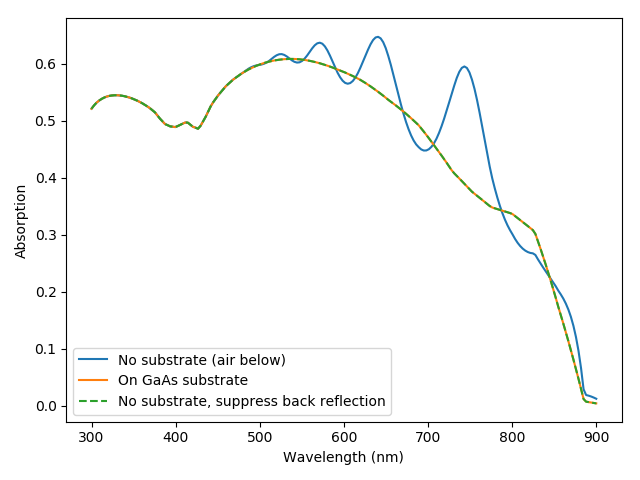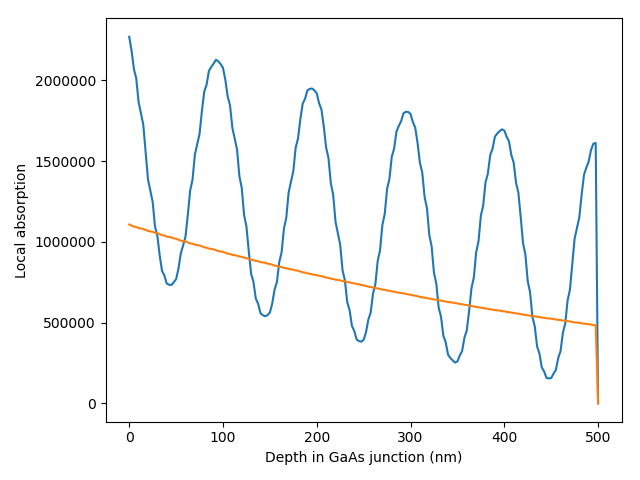# Looking at the effect of substrate and the no_back_reflexion option in the TMM solver¶```# TMM and RCWA solvers can take into account the presence of a substrate if it is specified in the solar_cell object.
# This example illustrates this for the TMM solver.

from solcore import material
from solcore import si
import matplotlib.pyplot as plt
import numpy as np
from solcore.solar_cell import default_GaAs, SolarCell, Layer, Junction
from solcore.solar_cell_solver import solar_cell_solver
from solcore.absorption_calculator import OptiStack

T = 298

GaAs = material('GaAs')(T = T)

thin_GaAs = SolarCell([Layer(material = GaAs, width=si('500nm'))])

GaAs_on_substrate = SolarCell([Layer(material = GaAs, width=si('500nm'))], substrate = GaAs)

wl = si(np.linspace(300, 900, 200), 'nm')

# Thin solar cell, no substrate - will get significant absorption enhancement from reflection at the GaAs/air interface at the back
# MUST specify no_back_reflexion = False, so that Solcore does not automatically suppress reflections from the back
# (currently, the default setting in solcore is to suppress reflections from the back, so no_back_reflexion = True
solar_cell_solver(thin_GaAs, 'optics', user_options={'wavelength': wl, 'optics_method': 'TMM', 'no_back_reflexion': False})
z_pos = np.linspace(0, thin_GaAs.width, 201)
profiles_thin = thin_GaAs.absorbed(z_pos)
# Same thin solar cell, but now on a GaAs substrate. In this case, we get the same result whether or not we specify
# no_back_reflexion to be True or False, since with a GaAs on GaAs cell we don't get any reflection at the back interface anyway
solar_cell_solver(GaAs_on_substrate, 'optics', user_options={'wavelength': wl, 'optics_method': 'TMM'})
profiles_thick = GaAs_on_substrate.absorbed(z_pos)

plt.figure()
plt.plot(wl * 1e9,  thin_GaAs.layer_absorption)
plt.plot(wl * 1e9,  GaAs_on_substrate.layer_absorption)

# Now we consider the thin solar cell without substrate again but ask Solcore to suppress back reflections. We must also
# ask Solcore to recalculate the absorption, otherwise it will just use the results calculated above which are already
# in the thin_GaAs object
# What no_back_reflexion = True actually does is add a highly absorbing layer based on the final layer in the stack so that
# nothing is reflected.

solar_cell_solver(thin_GaAs, 'optics', user_options={'wavelength': wl, 'optics_method': 'TMM', 'no_back_reflexion': True,
'recalculate_absorption': True})

plt.plot(wl * 1e9,  thin_GaAs.layer_absorption, '--')
plt.legend(labels=['No substrate (air below)', 'On GaAs substrate', 'No substrate, suppress back reflection'])
plt.xlabel("Wavelength (nm)")
plt.ylabel("Absorption")
plt.show()
# we can see that, correctly, the results for the cell with an explicitly specified GaAs substrate and for the thin
# cell purposely suppressing back reflections are the same, while the thin cell with back reflections shows thin-film
# oscillations in the absorption spectrum.

# Let's look at what the absorption profile looks like...

# absorption profile around 750 nm incident wavelength:
plt.figure()
plt.plot(z_pos*1e9, profiles_thin[:,150])
plt.plot(z_pos*1e9, profiles_thick[:,150])
plt.xlabel("Depth in GaAs junction (nm)")
plt.show()
```